Math Olympiad Test: Probability- 1

# Math Olympiad Test: Probability- 1

Test Description

## 10 Questions MCQ Test Mathematics Olympiad for Class 9 | Math Olympiad Test: Probability- 1

Math Olympiad Test: Probability- 1 for Class 9 2022 is part of Mathematics Olympiad for Class 9 preparation. The Math Olympiad Test: Probability- 1 questions and answers have been prepared according to the Class 9 exam syllabus.The Math Olympiad Test: Probability- 1 MCQs are made for Class 9 2022 Exam. Find important definitions, questions, notes, meanings, examples, exercises, MCQs and online tests for Math Olympiad Test: Probability- 1 below.
Solutions of Math Olympiad Test: Probability- 1 questions in English are available as part of our Mathematics Olympiad for Class 9 for Class 9 & Math Olympiad Test: Probability- 1 solutions in Hindi for Mathematics Olympiad for Class 9 course. Download more important topics, notes, lectures and mock test series for Class 9 Exam by signing up for free. Attempt Math Olympiad Test: Probability- 1 | 10 questions in 10 minutes | Mock test for Class 9 preparation | Free important questions MCQ to study Mathematics Olympiad for Class 9 for Class 9 Exam | Download free PDF with solutions
 1 Crore+ students have signed up on EduRev. Have you?
Math Olympiad Test: Probability- 1 - Question 1

### The probability of an impossible event is:

Detailed Solution for Math Olympiad Test: Probability- 1 - Question 1

The definition of probability is the degree to which something is likely to occur. An impossible event is an event with no chance of occurring.
Hence, the probability of an impossible event is zero.

Math Olympiad Test: Probability- 1 - Question 2

### Two coins are tossed simultaneously. The probability of getting at least one head is:

Detailed Solution for Math Olympiad Test: Probability- 1 - Question 2

On tossing two coins, following outcomes can be observed: {(H, H),(T, T),(H, T),(T, H)}
Therefore, there are total of 4 possible outcomes
Now, favourable outcomes are {(H,H),(H,T),(T,H)}
Therefore, no. of favourable outcomes are 3
Hence, P(Getting at least one head) = 3/4.

Math Olympiad Test: Probability- 1 - Question 3

### In a class, 20 students failed in a certain examination. If the no. of passed students is 80 and 1 student is selected at random, then the probability that the student has passed in the exam is:

Detailed Solution for Math Olympiad Test: Probability- 1 - Question 3

P (student passed in the examination)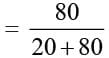= 0.8.

Math Olympiad Test: Probability- 1 - Question 4

A dice is rolled twice, and the outcomes are noted down. The probability of getting even no. as a sum is:

Detailed Solution for Math Olympiad Test: Probability- 1 - Question 4

P (drawing sum as even number)
= 18/36 = 1/2

Math Olympiad Test: Probability- 1 - Question 5

The probability of getting odd number as a unit place digit is:

Detailed Solution for Math Olympiad Test: Probability- 1 - Question 5

1, 3, 5, 7 and 9 are odd numbers
∴ Required probability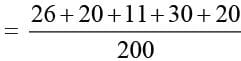= 107/200.

Math Olympiad Test: Probability- 1 - Question 6

A coin is tossed 1000 times, if the probability of getting a tail, 3/8 how many times head is obtained?

Detailed Solution for Math Olympiad Test: Probability- 1 - Question 6

Tail is obtained (3/8 × 1000) times = 375 times.
∴ Head is obtained (1000 – 375) = 625 times.

Math Olympiad Test: Probability- 1 - Question 7

The distribution of marks of 90 students are as follows: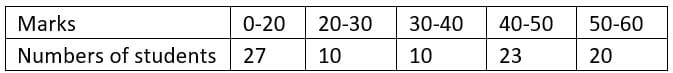The probability that a student obtained 40 or more marks is:

Detailed Solution for Math Olympiad Test: Probability- 1 - Question 7

P (student getting 40 or more marks)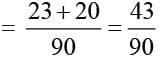Math Olympiad Test: Probability- 1 - Question 8

One card is drawn from a well shuffled deck of 52. What is  the probability of drawing a red card?

Detailed Solution for Math Olympiad Test: Probability- 1 - Question 8

P (getting a red card) = 26/52 = 1/2

Math Olympiad Test: Probability- 1 - Question 9

There are 36 students in a class of whom 20 are boys and remaining are girls. What is the probability that a student chosen is a girl?

Detailed Solution for Math Olympiad Test: Probability- 1 - Question 9

P (student chosen is girl)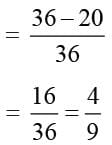Math Olympiad Test: Probability- 1 - Question 10

Two dice are thrown simultaneously. What is the probability of getting a doublet?

Detailed Solution for Math Olympiad Test: Probability- 1 - Question 10

(1, 1), (2, 2), (3, 3), (4, 4), (5, 5), (6, 6) are the doublets
∴ P (getting a doublet) = 6/36 = 1/6.

## Mathematics Olympiad for Class 9

1 videos|43 tests
 Use Code STAYHOME200 and get INR 200 additional OFF Use Coupon Code
Information about Math Olympiad Test: Probability- 1 Page
In this test you can find the Exam questions for Math Olympiad Test: Probability- 1 solved & explained in the simplest way possible. Besides giving Questions and answers for Math Olympiad Test: Probability- 1, EduRev gives you an ample number of Online tests for practice

## Mathematics Olympiad for Class 9

1 videos|43 tests